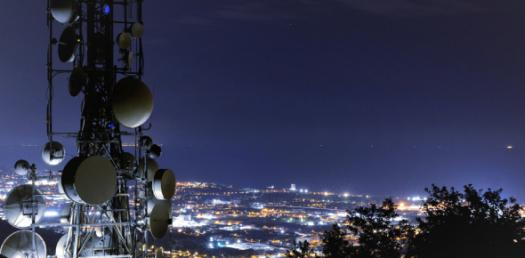# Trivia Questions On Antenna Theory And Wave Propagation! Quiz

15 Questions | Total Attempts: 316Settings• 1.
Top loading is used in an antenna in order to increase its ______.
• A.

Practical height

• B.

Beamwidth

• C.

Effective height

• D.

Input capacitance

• 2.
A horizontal antenna is _____.
• A.

Vertically polarized

• B.

Horizontally polarized

• C.

Centrally polarized

• D.

Circularly polarized

• 3.
All elements in a beam _____ antennas are in line
• A.

Collinear

• B.

Yagi

• C.

• D.

Log-periodic

• 4.
Which does not use vertical polarization antenna?
• A.

• B.

• C.

Mobile Communications

• D.

Satellite Communications

• 5.
Lightning rods must be mounted a top structure not less than ___ above the highest point.
• A.

10 cm

• B.

20 cm

• C.

30 cm

• D.

40 cm

• 6.
_____ is an antenna with a number of half-wave antenna on it.
• A.

Antenna array

• B.

Tower

• C.

Omni-directional

• D.

Rhombic

• 7.
Which antenna radiates an omnidirectional pattern in the horizontal plane with vertical polarization?
• A.

Marconi antenna

• B.

Discone antenna

• C.

Horn antenna

• D.

Helical antenna

• 8.
An antenna with very high gain and very narrow beamwidth
• A.

Helical antenna

• B.

Discone antenna

• C.

Horn antenna

• D.

Parabolic dish antenna

• 9.
_____ is the horizontal pointing angle of an antenna
• A.

Azimuth

• B.

Angle of elevation

• C.

Right angle

• D.

Beamwidth

• 10.
What determines antenna polarization?
• A.

The frequency of the radiated wave

• B.

The direction of the radiated wave

• C.

The direction of the magnetic field vector

• D.

The direction of the electric field vector

• 11.
A dipole antenna requires to be fed with 20 kW of power to produce a given signal strength to a particular distant point. If the addition of a reflector makes the same field strength available with an input power of 11kW. What is the gain in dB, obtained by the use if the reflector. (Gain referred to this particular dipole).
• A.

4.75

• B.

2.6

• C.

1.81

• D.

4.24

• 12.
What is the estimated medium wind loading in the Philippines for antenna tower design?
• A.

300 kph

• B.

100 kph

• C.

200 kph

• D.

250 kph

• 13.
• A.

Omnidirectional/vertical polarized

• B.

Unidirectional

• C.

Bidirectional

• D.

Figure of eight

• 14.
_____ is an open-ended slot antenna
• A.

Helical antenna

• B.

Rhombic antenna

• C.

Notch antenna

• D.

Cassegrain antenna

• 15.
Which antenna is a properly terminated?
• A.

Marconi

• B.

Rhombic

• C.

Dipole

• D.

Yagi-Uda

Related TopicsBack to top